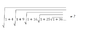# Finding limit of infinite term

phasair

## Homework Statement

The problem is not homework, just something that has been bothering me. It's in picture form, in the attachment.
I'm an undergraduate in math, and this type of convergence is a bit unknown to me. What I've seen so far are normal sequences and series, and power series.
This however, is different, because there is no 'first' term, no 'beginning', so I don't really know how to approach this.

## The Attempt at a Solution

The equation I've found that describes this term is:

f(x)^2 = 1 + (x^2)f(x+1)

In this case, we would be looking for f(2). However, that equation doesn't contain enough information to solve it, because there are two free variables.

Any help would be appreciated.

#### Attachments

•1345785316964.png
5 KB · Views: 462

Millennial
You found a functional equation. You only need one particular value of the function. For example, what is f(0)?
Note: You have an identity $f(x)=f(-x)$ here. Use it.

Last edited: